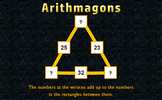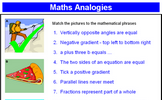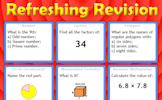# Expanding and factorising

### Term 1 starting in week 7 :: Estimated time: 2 weeks

• Expand and factorise with a single bracket (review)
• Expand binomials (review)
• Solve equations equal to zero
• Solve quadratic equations by factorisation

For higher-attaining pupils:

• Solve complex quadratic expressions by factorisation

This page should remember your ticks from one visit to the next for a period of time. It does this by using Local Storage so the information is saved only on the computer you are working on right now.

## Lesson Starters

Here are some suggestions for whole-class, projectable resources which can be used at the beginnings of each lesson in this block.

### 1st Lesson#### Arithmagons

This lesson starter requires pupils to find the missing numbers in this partly completed arithmagon puzzle.

### 2nd Lesson#### Lemon Law

Change the numbers on the apples so that the number on the lemon is the given total.

### 3rd Lesson#### Analogies

Make more of analogies to help remember mathematical concepts.

### 4th Lesson#### Big Order

Estimate or calculate then put the large numbers in order of size.

### 5th Lesson#### Refreshing Revision

It is called Refreshing Revision because every time you refresh the page you get different revision questions.

### 6th Lesson#### Cube Calendar

What numbers should be on each face of the two cubes to make this perpetual calendar?

Some of the Starters above are to reinforce concepts learnt, others are to introduce new ideas while others are on unrelated topics designed for retrieval practice or and opportunity to develop problem-solving skills.

White Rose ResourcesEnd of block assessments provide a quick progress check at the end of each block of learning to make sure students have understood the content covered. This Scheme of Learning was produced by White Rose Maths and is used here with permission granted on 30th June 2021.For All: